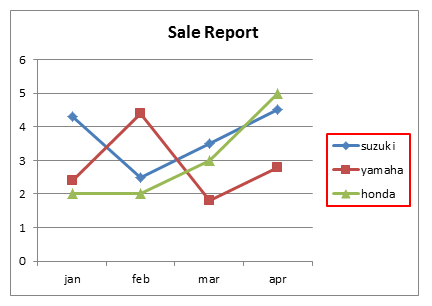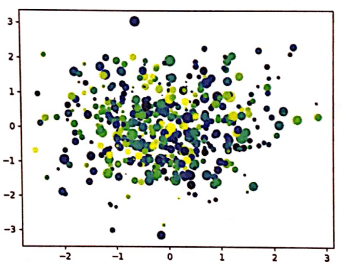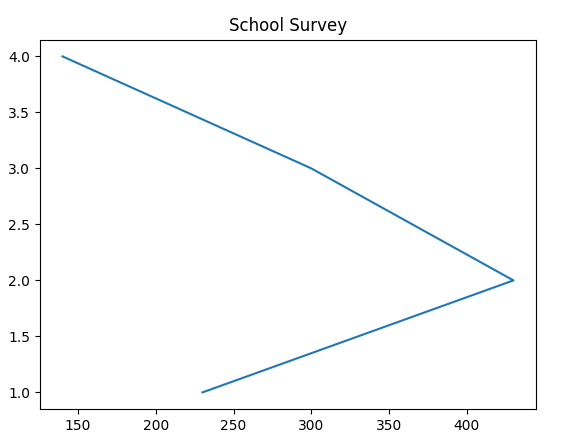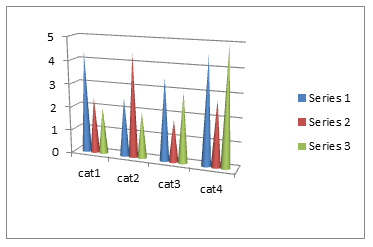# Matplotlib Plotting with pyplot MCQ | Data Visualization MCQThis post contains Multiple Choice based Questions with answers for Matplotlib Plotting with pyplot MCQ. These objective questions with answers are not only helpful for those students preparing for CBSE IP Class 12 Matplotlib Plotting with pyplot MCQ but also for all those students who are practicing and learning Python Programming specially Data Handling and Visualization using Python Matplotlib Plotting with pyplot .

You will get different categories of Questions for all the concept of Python Matplotlib Plotting with pyplot objective questions-

Assertion and Reason MCQs
Case Study Based MCQs
Find The Output Based MCQs
Concept Based MCQs

#### Matplotlib Plotting with pyplot MCQ SET-1 (Q1-Q25)

1. Which of the following does not visualize data?
a. Charts
b. Maps
c. Shapes
d. Graphs

c. Shapes

1. Which of the following type of chart is not supported by pyplot?
a. Histogram
b. Boxplot
c. Pie
d. All are correct

D. All are correct

1.  In the given chart, box surrounded with red border is calleda. Data series
b. Chart Title
c. Markers
d. Legend

d. Legend

1. To display histogram with well-defined edge we can write

a. df.plot( type = ‘hist’, edge = ‘red’)
b. df.plot( type = ‘hist’, edgecolor = ‘red’)
c. df.plot( type = ‘hist’, line = ‘red’)
d. df.plot(type = ‘hist’, linecolor = ‘red’)

b. df.plot( type = ‘hist’, edgecolor = ‘red’)

1. plot which is used to given statistical summary is

a. Bar
b. Line
c. Histogram
d. Box plot

d. Box plot

1. Which of the following is not the parameter of pyplot’s plot() method?

a. Marker
b. Lineheight
c. Linestyle
d. Color

b. Lineheight

1. To compare data we can use ____ chart

a. Line
b. Bar
c. Pie
d. Scatter

b. Bar

1. Which of the following chart element is used to identify data series by its color patterns?

a. Chart title
b. Legend
c. Marker
d. Data labels

b. Legend

1. Identify the following chart’s typea. Scatter Chart
b. Bubble Chart
c. Pie Chart
d. Frequency Polygon

a. Scatter Chart

1. Look at the following graph and select appropriate code to obtain this output. (Please assume that pandas and matplotlib is already imported)a. zone=[1,2,3,4]
schools = [230,430,300,140]
plt.plot(zone, school, ‘School Survey’)
plt.show()

b. zone=[1,2,3,4]
schools = [230,430,300,140]
plt.plot(schools,zone, ‘School Survey’)
plt.show()

c.  zone=[1,2,3,4]
schools = [230,430,300,140]
plt.plot(zone, school)
plt.title(“School Survey”)
plt.show()

d. zone=[1,2,3,4]
schools = [230,430,300,140]
plt.plot(schools,zone)
plt.title(“School Survey”)
plt.show()

```d.  zone=[1,2,3,4]
schools = [230,430,300,140]
plt.plot(schools,zone)
plt.title("School Survey")
plt.show()```

1. Which of the following is incorrect regarding Data Visualization?

a. Data visualization can be done using Matplotlib library in python.
b. Visualizing large and complex data does not produce effective results.
c. Data visualization is immensely useful in data analysis.
d. Decision makers use data visualization to understand business problems easily and build strategies.

b. Visualizing large and complex data does not produce effective results.

1. Matplotlib is _____ plotting library

a. 1D
b. 2D
c. 3D
d. All of above

b. 2D

1. Data _____________ refers to graphical representation of data.

a. Visualization
b. Analysis
c. Plotting
d. Handling

a. Visualization

1. consider the code given below:
plt.bar(cities, population, color = [‘r’,’g’,’b’,’m’])
what will be color of last bar?

a. Magenta
b. Green
c. Blue
d. Black

a. Magenta

1.  Following chart is type ofa. Line Chart
b. Bar chart
c. Histogram
d. Scatter chart

b, Bar Chart

1. Raj has written following code to create a bar chart-

Import matplotlib.pyplot as plt
weekdays  = [‘sun’,’mon’,’tue’]
sale = [1234,3312,2541]
plt.bar(weekdays, sale)

But when executing this code he is not able to display any chart. What would you suggest him to execute code and display chart successfully?

a. Instead of using bar() he should use plot() method
b. He should add show() function at the end of code
c. He should use numpy array to store weekdays and sale data
d. No suggestion required

b. He should add show() function at the end of code

1. The interface of Matplotlib used for data visualization is

a. Seaborn
b. Anaconda
c. matlab
d. pyplot

d. pyplot

1. the best suitable real life example of data visualization is

a. Percent of population of by age group in India
c. Solar system drawing
d. Both a and b
e. All of the above

d. both a and b

1. Which of the following is correct code to plot line chart with dotted linestyle?

a. Plt.plot(x,y)
Plt.linestyle  = “dotted”
Plt.show()

b. Plt.plot(x,y)
Plt.linestyle(“dotted”)
Plt.show()

c. Plt.plot(x,y, linestyle = “dotted”)
Plt.show()

d. All are correct

```c.  Plt.plot(x,y, linestyle = “dotted”)
Plt.show()```

1.  in the given chart, box surrounded with red border is calleda. Label
b. X label
c. Y label
d. Legend

b. X label

1. Which of the following is correct syntax to create histogram with bins specified?

a. Plt.hist(x, bins=10)
b. Plt.hist(x,bins = [10,11,12,13,14])
c. Plt.hist(x, bins = range(10,15))
d. All are correct

All are correct

1. If you install python IDLE, matplotlib is installed automatically.

a. True
b. False

b. False

1. The command to install Matplotlib library in python is

a. Install pip matplotlib
b. Install matplotlib
c. Pip matplotlib
d. Pip install matplotlib

d. Pip install matplotlib

1.  in the given chart, box surrounded with red border is calleda. Label
b. X label
c. Y label
d. Legend

c. Y label

1. Select the correct statement to display horizontal box plot

a. Plt.box(data, vert=False)
b. Plt.box(data, horiz=True)
c. Plt.boxplot(data, vert = False)
b. Plt.boxplot(data, horiz = True)

c. Plt.boxplot(data, vert = False)

#### MCQ of Class 12 IP (Informatics Practices)

Data Handling and Visualization Notes : https://techtipnow.in/data-handling-pandas-data-visualization-notes/

Database Query Using SQL Notes : https://techtipnow.in/class-12-ip-database-query-using-sql-notes/

Introduction to Computer Network : https://techtipnow.in/introduction-to-computer-network-notes/

Societal Impact Notes : https://techtipnow.in/societal-impact-notes-societal-impact-ip-class-12/

Previous Year Question Paper IP Class 12: https://techtipnow.in/previous-year-question-paper-ip-class-12/

CBSE Sample Papers : https://techtipnow.in/ip-sample-paper/

Practice Sample Paper IP : https://techtipnow.in/ip-sample-paper-class-12-with-solutions/

NCERT Text Book Solution IP Class 12: https://techtipnow.in/ncert-solution-ip-class-12/

Sumita Arora Textbook Solution : https://techtipnow.in/sumita-arora-textbook-solution-information-practices-xii/

Python Pandas Series MCQ : https://techtipnow.in/python-pandas-series-mcq/

Python DataFrame MCQ : https://techtipnow.in/python-pandas-dataframe-mcq/

Plotting with PyPlot MCQ : https://techtipnow.in/python-data-visualization-mcq/

Societal Impact MCQ : https://techtipnow.in/python-data-visualization-mcq/

SQL MCQs : https://techtipnow.in/sql-mcq-class-12/

MySQL Functions MCQ : https://techtipnow.in/mysql-functions-mcq/

IP Class 11 Notes : https://techtipnow.in/ip-class-11-notes/

IP Class 11 MCQs : https://techtipnow.in/ip-class-11-mcq/

NCERT Solution Class 11: https://techtipnow.in/ncert-textbook-solution-ip-class-11/

### 10 thoughts on “Matplotlib Plotting with pyplot MCQ | Data Visualization MCQ”

1.Very good

1.Thanks for appreciation..Keep learning!!

2.Thank u sir for helping us so much

3.it was very useful thank u

4.good

5.Sir in cuet,questions will be like that??

error: Content is protected !!